JEE  >  Theory & Procedure, Friction

# Theory & Procedure, Friction Notes | Study Additional Documents and Tests for JEE - JEE

## Document Description: Theory & Procedure, Friction for JEE 2022 is part of Documents for Additional Documents and Tests for JEE preparation. The notes and questions for Theory & Procedure, Friction have been prepared according to the JEE exam syllabus. Information about Theory & Procedure, Friction covers topics like and Theory & Procedure, Friction Example, for JEE 2022 Exam. Find important definitions, questions, notes, meanings, examples, exercises and tests below for Theory & Procedure, Friction.

Introduction of Theory & Procedure, Friction in English is available as part of our Additional Documents and Tests for JEE for JEE & Theory & Procedure, Friction in Hindi for Additional Documents and Tests for JEE course. Download more important topics related with Documents, notes, lectures and mock test series for JEE Exam by signing up for free. JEE: Theory & Procedure, Friction Notes | Study Additional Documents and Tests for JEE - JEE
 1 Crore+ students have signed up on EduRev. Have you?

Objectives

• To study the relationship between force of limiting friction and normal reaction.
• To find the co-efficient of friction between a block and a horizontal surface.

Theory

When one body makes an attempt to slide over another body, an opposing force called the force of friction arises as a reaction to the applied force, and acts in the opposite direction.

Static friction is the type of friction that exists when two objects touch each other at rest. It has a limiting value called limiting friction, which is equal to the least force required to move the body from rest. When the external force F is increased, a stage comes when the body is just at the verge of moving. At this stage, the force of friction is at the maximum, and is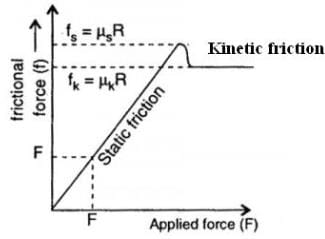called limiting friction.

It is found experimentally that limiting frictional force,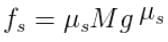where is called the coefficient of static friction. It is a dimensionless constant, but depends on the nature of surfaces in contact.

When the applied force, F, is increased further (beyond the limiting frictional force), the body begins to move, then the force opposing the motion is called the kinetic or sliding friction. The kinetic friction is less than the limiting friction. The force of kinetic friction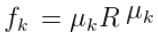where is called the coefficient of kinetic friction.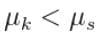The Laws of Static Friction are:

i.  The magnitude of limiting friction depends upon the nature and state of polish of the two surfaces in contact.
ii. The magnitude of limiting friction is independent of area and shape of surfaces in contact as long as the normal reaction    remains the same.
iii. The magnitude of limiting friction 'F' is directly proportional to normal reaction 'R' between the two surfaces in contact.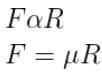μ where is called the coefficient of friction which is a measure of friction based on the type of material that are in contact.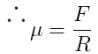The coefficient of friction,

A graph can be drawn with normal reaction, R along X axis and limiting friction, F along Y axis and it is a straight line.

Learning Outcomes:

• Students understand underlying concepts of friction.
• They learn about limiting friction, normal reaction and its relationship.
• They understand coefficient of friction.

Materials Required

• Wooden block
• 50 or 20 g slotted weights
• Horizontal plane (table top) fitted with a frictionless pulley at one end
• Weight box
• Spring balance

Real Lab Procedure

• Find the mass of the wooden block by using a spring balance and place it on the table fitted with a frictionless pulley at one end.
• Tie one end of a thread with the hook of the wooden block and pass it over the pulley.
• Tie the other free end of the thread to the weight hanger and let it hang vertically.
• Increase the mass, till the block just starts sliding when tapping the table.
• Note the total mass added to the weight hanger and multiply it with acceleration due to gravity, g to get the total weights added and record them in the observation table.This gives the force of limiting friction, F.
• The normal reaction, R is given by the mass, M of the wooden block multiplied by acceleration due to gravity, g.
• Repeat the experiment with different masses on the block and in each case is calculated and it is found to be a constant for  the given pair of surfaces.
• Plot a graph taking normal reaction, R along X axis and limiting friction, F along Y axis. The graph will be a straight line sloping upwards.
• The coefficient of friction is also calculated by measuring the slope of the graph.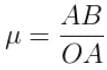Simulator Procedure (As performed through the Online labs)

• Select the material of the block from the 'Block material’ drop down list
• Select the material of the surface to perform the experiment from the ‘Surface material' drop down list.
• Use the ‘Block Weight’ slider to change the weight of the block.
• A thread is fixed at one side of the block and passes over a pulley.
• Use the ‘Hanging Weight’ slider to change the weight attached at the other end of the thread.
• The block moves towards right according to the addition of mass attached at the other end.
• A graph is plotted automatically with normal reaction along X axis and limiting friction along Y axis.
• The mass of the block and attached weights are noted.
• Now, calculations are done as per the observation column and the coefficient of friction of the block can be found out.
• Enable the ‘Show result’ checkbox to view the limiting friction, normal reaction, and coefficient of friction of the selected block.
• Click on the ‘Reset’ button to redo the experiment.

Observations

Weight of the wooden block, W =...................g wt

Table for finding coefficient of friction

 No of Observations Weight on wooden blockW(g wt) Normal ReactionR=W+w(g wt) Total weights on weight hanger=Limiting frictionF(g wt) Coefficient of friction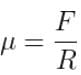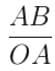From graph, coefficient of friction can be calculated as ,  =--------

## Result

• Since F/R is a constant, the value of limiting friction is directly proportional to normal reaction.
• By calculation, Coefficient of fiction between given surfaces, µ =-------.
• From graph, Coefficient of fiction between given surfaces, µ =-------.
The document Theory & Procedure, Friction Notes | Study Additional Documents and Tests for JEE - JEE is a part of the JEE Course Additional Documents and Tests for JEE.
All you need of JEE at this link: JEE

## Additional Documents and Tests for JEE

11 videos|159 docs|14 tests
 Use Code STAYHOME200 and get INR 200 additional OFF

## Additional Documents and Tests for JEE

11 videos|159 docs|14 tests

Track your progress, build streaks, highlight & save important lessons and more!

,

,

,

,

,

,

,

,

,

,

,

,

,

,

,

,

,

,

,

,

,

,

,

,

;